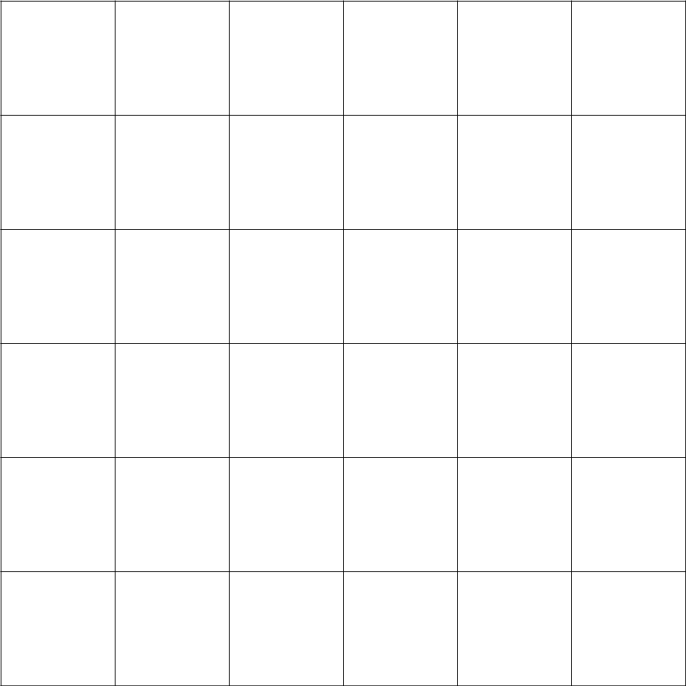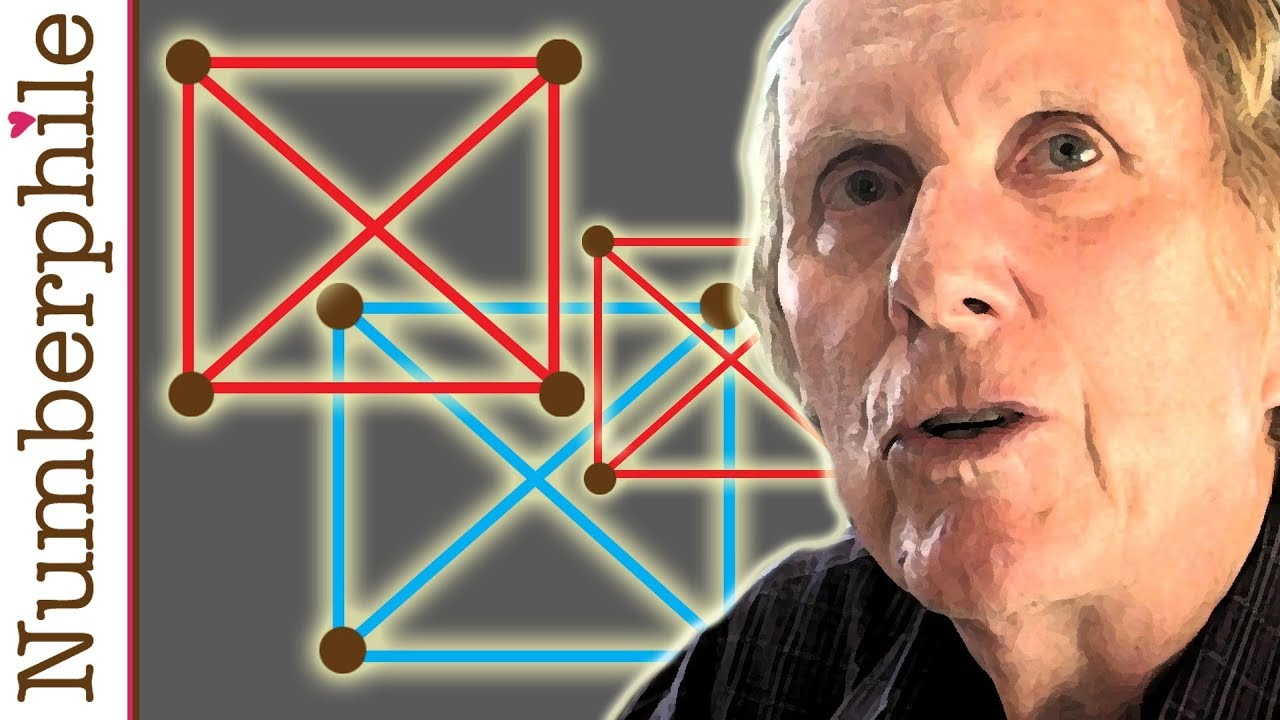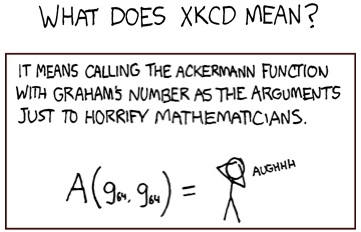# Do we see in 3 dimensions?

Hi,
its been quite long time since I wrote about dimensions. Just a quick recap since people do not click on links much: 0th dimension is point, 1st dimensions is line, 2nd dimension is plane and 3rd dimension is space. We are talking about spatial dimensions, another thing is atime dimension, but that is not important here.

So I got into this argument if humans see in 3 dimensions or not, I got no clear conclusion though so I am just going to discuss it here. We live in 3 spatial dimensions, proof, cubes exist. How do we see though? We can see cubes but I would argue that we see in 3rd dimension.

1. Retina does not capture depth, it is 2 dimensional detector so we simply can not have a 3 dimensional vision.
2. We have two eyes though, lot of people seem to like to point this out. With second eye you have what is called a stereoscopic vision.. the second eye has little bit different point of view and when brain combines it stuff gets depth. This does not mean that we see in 3D though. Still it is just two 2 dimensional pictures put together. Brain interprets this picture as 3 dimensional and based on experience judges distance. Also colors and shades can help us with that.This is stereoscopic picture, disalign your eyes and match the pictures so you see 3 of them.

3. You can draw anything you see on piece of paper and it will be exact representation of what you see, all angles same and so on. This I think would be impossible if you actually wanted to transfer 3D image to 2D plane because by definition 3D space is made up of infinite 2D planes put on top of each other. Same as you can not take infinite picture and store it in 1D line.
4. If we could see in 3D we would see all angles as they actually are. If you look into corner you do not see 90° angles though because you remember the way that walls work you know that they are there.

Dragallur

# One week of mathematics, #4

Hi,
it was thursday, the last whole day on math course. It was snowing all the time if I remember correctly. Again we had two morning lectures and then one evening.

The first one was one of the best there, it was about combinatorics and random walk across square grid. I mean, really cool, lets see:Take a grid.
Now imagine you want to get from top left point to right down corner at the least number of steps. In those steps you always want to move to right or down, so every time you get closer. You can only move across black lines, now lets look how many ways there are.

OK, look at the next picture. There are clearly too many ways to get there,we need some bette way to do this.You start at “1”. There are only two options to go. You can go vertically or horizontaly. So there is only one way to get to point 2 and 8. When you want to get to point 9 you could either go through 2 or 8 so there are two ways to get there, as this we can fill in whole grid.You can see that the numbers always add up and there are 924 ways to get to other side. Also the grid is symmtrical. I could also say that you can not step on some point. Then you will add up from left top to right bottom but the number on that step would be zero because no way would come through the spot. Or also you could say that I have to go through one spot. Well I would make two rectangle/squares from this table and first take the ways to get to the spot and then from the spot to the end, then I would multiply both numbers to get the number of ways, one can get there.

Such a random walks can also be extended to higher dimensions, it gets kind of mess but it would be fun to do it in giantic 5 dimensional cube, I mean the ways would increase extremely, like a lot.

After this amazing stuff we had lecture which at the start seemed to be pretty good. It was about usage of excel in mathematics, particularly matrices.

The guy probably thought that we know a lot more and I do not think that there were many (maybe any) people who understood him. When we asked him something, he just said to us that we should read English Wikipedia that he wont waste time there. He was asshole.

During the rest of the day I felt like walking zombie. I had headache, I was sick a lot and there was one more lecture in the evening about conics, but it was too difficult for me to follow it and I was way too tired and went to sleep at around 20:30.

Dragallur

# Dimensions: Short Expansion

Hi,
here it comes, here it goes, again seemingly very favorite topic about dimensions. Last time I wrote about imagining higher dimensions and how they work and that we have 3+1 dimensions. 3 are space dimensions and one time dimension. Actually I should have added that there is and option of much more dimensions. Thanks to Joseph from Rationalising The Universe reminding me of this through his post which I recommend you to read.

Fine if you read his post you know that I want to talk about dimensions that can exist in string theory.

So there are two main theories: Theory of Relativity and Quantum theory.
What we know for sure that both are wrong. This is because TOR is not able to predict stuff on small scales and QT on large scales while they are both very good on their fields.
Then there is third guy called String theory. This theory says that on very small scale fundamental particles are one dimensional strings, which mean lines and when you are looking at them “from above” they look like particles.

String theory predicts a lot of dimensions on extremely small scales (check Josephs post to read about gravity etc.).
There are two most important ST theories. Boson and Superstring theories. The first one predicts 26 dimensions and second 10, now choose :D.
The thing is that actually for some stuff it is much better to have more dimensions.
Since for me it is so extremely complex I can just advice you for wiki page for start.
Still String theory is just theory as are others and I am totally looking forward to Theory of Everything!
Dragallur

# Turn your brain into black hole

Hi,
here it goes, here it comes! Today I want to write about Graham’s number which is very interesting number and highest number which has some usage in mathematics.

Ok first of all, what is its purpose?
Imagine square where all vertices are connected. You can connect them by two colors, blue and red. You are trying to avoid one special pattern whichyou can see behing the mathematician Ron Graham who invented this number about which I will talk in a while. So those points can not be connected by single color the thing is that when you increase the dimension it is little harder (you already now everything about dimensions if you read my last post).When you have 3rd dimension as you can see on the right there are 8 vertices.
This means that there are 28 lines between those dots.
Yes it is possible to avoid the special configuration. When you continue to fourth dimension you can still do it but here comes the problem. In fourth dimension there are 2^`120 possible ways to color this cube. This is such a large number that no computer can solve it, in decent amount of time. Now you need to make some better way to solve it. Through some better way which I dont know mathematicians got up to 13th dimension, now they dont know maybe it is possible maybe not but the number when we are COMPLETELY sure that it is not possible is Graham’s number.

To show you how large is Graham’s number I first of all have to explain Knuth’s up-arrow notation.
3+3+3=3*3
3*3*3=3^3
3^3=3↑3=27
That’s about it when you have one arrow. It gets better when you have two:
3↑↑2=3^3=27 , easy.
3↑↑3=3^3^3=3^27=over 7 trillions$\begin{matrix} a\uparrow\uparrow b & = {\ ^{b}a} = & \underbrace{a^{a^{{}^{.\,^{.\,^{.\,^a}}}}}} & = & \underbrace{a\uparrow (a\uparrow(\dots\uparrow a))} \\ & & b\mbox{ copies of }a & & b\mbox{ copies of }a \end{matrix}$
Lets see three of them:
2↑↑↑2=2↑↑(2↑↑2)=2↑↑4=2^2^2^2=2^2^4=2^16=65536
2↑↑↑3=2↑↑(2↑↑(2↑↑2)=2↑↑65536$\begin{matrix} a\uparrow\uparrow\uparrow b= & \underbrace{a_{}\uparrow\uparrow (a\uparrow\uparrow(\dots\uparrow\uparrow a))}\\ & b\mbox{ copies of }a \end{matrix}$
Easy it continues and it gets hella huge.
So when I found out that Graham’s number needs arrow notation to be written I was like: “Easy, there will be few arrows, arrow notation is such extreme tool.”
Then after I found out how big is Graham’s number I was just like: “Oops!” You will se why.
So lets define the number g1
g1=3↑↑↑↑3
=3↑↑↑(3↑↑↑3)
=3↑↑↑(3↑↑(3↑↑3)

=3↑↑↑(3↑↑(3↑(3↑3)
=3↑↑↑(3↑↑(3↑27) i think that this is enough for ilustration.
now lets define g2=3↑↑↑↑↑↑↑…3 number of arrows equals to g1

Surprised? We are not even in beginning.
g3=3↑↑↑↑…3 number of arrows equals to g2 … so you know the process, this continues up to g64 which is Graham’s number.

Ok this is extremely large number and to remind you it is number of dimensions, which means that the combinations are even much higher, not kidding.
The Graham’s number has real meaning but I am not really able to understand so if you want to check it, somewhere in the video Ron Graham explains it. Also Numberphile has some other videos about it so check them out.
Next time I will write about Ackermann function. After that you will get this XKCD joke:Dragallur

PS: I found lot of people trying to liken stuff to Graham’s number. Dont even try that, you wont get very close.

# Dimensions

Hi,
Here it goes, here it comes! Really long time (means few years) I was wondering about dimensions. It started when I was watching videos like: how to imagine higher dimensions, what are dimensions and so on. At the beginning I did not understand any of it.

As time went on I accidentally bumped into black holes and later on into wormholes (actually this post has some mistakes asfor  example it is saying that time is fourth dimension.) which should  connect space through higher dimensions and make a shortcut through Universe (picture is from here).

Ok first of all lets clear out some things. This post can have some mistakes, but I hope that there wont be any.
Second, fourth dimension is not really a time. The thing is that time does not work like space dimensions. Space dimensions also known as spatial dimensions, are the dimensions in which we can move forward, backwards, horizontaly, verticaly.. like in our world. Time is not so free, it runs just one direction and it is special type of dimension called the temporal dimension.
We live in 3+1 dimensional world.

Ok so what are those normal spatial dimensions? Well I will start with the most simple dimension, 0th dimension.On the picture you can see 0th dimension, pretty fun huh? It is point, and point has no volume by the way, this one is just enlarged. In 0th dimension you can not do anything, I am serious.

The problem is that if you lived in 0th dimension you would not be able to imagine 1th dimension because it would be like imagining new color which you never saw.
When it comes to creating higher dimensions ALWAYS you have to copy the lower dimension infinite amount of times. This means that if you want to have 1st dimension which is line you have to line up infinite amount of points from 0th dimension. As you probably know from math there is infinite possible places to place point on line.So here comes the 1st dimension which is line made up of infinite amount of dots. On line you can place all of the real numbers.
In the movie Flatland, which is fun movie you watch the line creatures to move back and forth because they live in 1st dimension.

I dont need to put any picture of second dimension, since you see it every day, on your computer, book on board in school.
To create 2nd dimension, again you need infinite amount of lines next to each other to create plane. Now 2nd dimension is pretty useful, for lot of stuff from: graphs, photos, any text, movies… you know I could continue for long time.So the standard object of 3rd dimension is cube. Again when you want to create it you need infinite amount of planes because they dont have any thickness.
Well now we are in our world but of course I can continue for the fourth dimension.

Ok the problem is with imagining 4th and higher dimensions on their dimension. By this I mean that I can easily draw on plane any dimension but it is just impossible to imagine 4th dimension since in our world we dont have space to expand it into. Over time I found some things that can help you anyway.In one episode of Futurama Doctor says: if you looked from fourth dimension to third you would see inside of this egg as when you look from third dimension to second and you can see egg’s inside. I would say that this is little mind bending. In fourth dimension there is direction in which you can move but from here we can not imagine the direction since it seems that we can already move anywhere.
Finally here comes the drawing of dimensions: Always the angle between new dimensions is 90 degrees. Inthird already you have to imagine it because if you would measure the angle on the plane you would have about 60 degrees. But it continues and the angle is still 90 even if it seems impossible. 5th dimensions is just expansion of all of those dots to another direction and so on and so on (the picture is from here).

Actually from plane you can see 3rd dimension with pretty neat trick that I found some time ago. On the next picture there is 3D object in 3D, 2D and 1D. To see the first one in 3D you have to look as you would look behind it so there are fourth of those objects on row. At one point those objects would be only three because two would be over each other. If you are precise enough you can match them well enough to see the picture in 3D.Well this is about it. (The last picture is from here.)